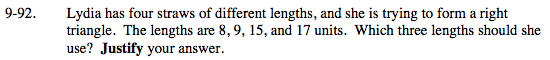### Home > MC2 > Chapter 9 > Lesson 9.3.2 > Problem9-92

9-92.The Pythagorean Theorem states that for any right triangle, the sum of the squares of the lengths of the legs is equal to the square of the length of the hypotenuse.

(leg 1)2 + (leg 2)2 = (hypotenuse)2

Square every length.

(8)2 = 64
(9)2 = 81
(15)2 = 225
(17)2 = 289

The lengths cannot be 8, 9, and 15 because: (8)2 + (9)2 ≠ (15)2.
Find the right combination of lengths that make the equation true.